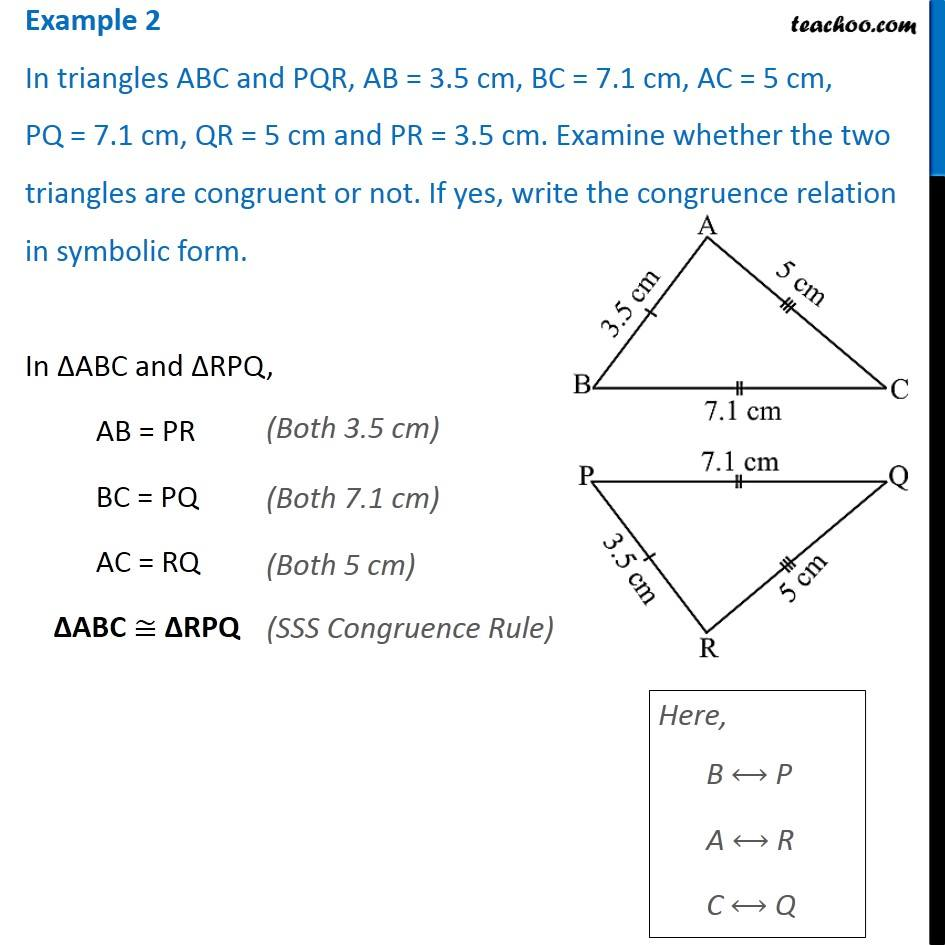1. Chapter 7 Class 7 Congruence of Triangles
2. Concept wise
3. SSS Congruency Criteria

Transcript

Example 2 In triangles ABC and PQR, AB = 3.5 cm, BC = 7.1 cm, AC = 5 cm, PQ = 7.1 cm, QR = 5 cm and PR = 3.5 cm. Examine whether the two triangles are congruent or not. If yes, write the congruence relation in symbolic form.In ∆ABC and ∆RPQ, AB = PR BC = PQ AC = RQ ∆ABC ≅ ∆RPQ